Your data analysis solution

# Factor analysis in Excel tutorial

2017-11-30

This tutorial will help you set up and interpretFactor Analysis (FA) in Excel using the XLSTAT software.
Not sure if this is the right multivariate data analysis tool you need? Check out this guide.

## Dataset for running a Factor Analysis

An Excel sheet with both the data and the results can be downloaded by clicking on the button below:

The data are from [Kendall M. (1975). Multivariate analysis. Griffin, London] and correspond to 48 applicants for a position in firm who have been judged on 15 variables:

• Form of letter of application
• Appearance
• Academic ability
• Likeability
• Self-confidence
• Lucidity
• Honesty
• Salesmanship
• Experience Drive
• Ambition Grasp
• Potential Keenness to join
• Suitability

Because many correlations between the variables are high, it was felt that the judge might be confusing some of the variables, or that some variables might be redundant. Therefore a factor analysis was conducted to determine the fewer underlying factors.

Several methods are available for computing factor analysis. XLSTAT default method is the Principal factor method applied iteratively. We will apply it here to generate seven factors, and we will do a varimax rotation to facilitate the interpretation of the results.

## Setting up a Factor Analysis in XLSTAT

After opening XLSTAT, select the XLSTAT / Analyzing data / Factor analysis commanD (see below).Once you've clicked on the button, the Factor analysis dialog box appears. Select the data on the Excel sheet.

The Observations labels are also selected in the corresponding field.In the Options tab we select the varimax option for the rotation that will be applied to the first two factors.The following options have been activated for the outputs and the charts.The computations begin once you have clicked on OK. The results will then be displayed.

## Interpreting the results of a Factor Analysis

The first results that are displayed are the summary statistics of the selected variables, and the correlation matrix between the variables. We can see that some of the correlations are quite high (0.883 for Grasp and Lucidity).

The standardized Cronbach's alpha is computed for the whole input table. An alpha of 0.914 means that there is some redundancy among the selected variables.

The reproduced and residual correlation matrices allow to verify if the factor analysis model is fine or not, and where it fails to reproduce correlations.

The next table shows the eigenvalues resulting from the factor analysis. We can see that with 4 factors we keep 75.5 % of the variability of the initial data.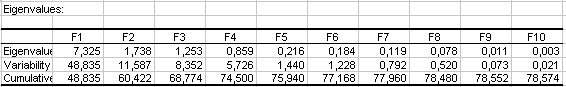Note: the eigenvalues displayed above are those obtained with the principal factors extraction method.

With the principal components analysis we would have obtained the following results: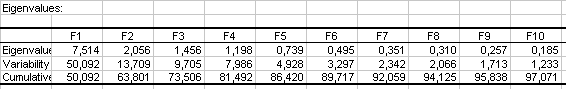Next, we can see that the varimax rotation has changed the way each factor explains part of the variance.

The varimax rotation makes the interpretation easier by maximizing the variance of the squared factors loadings by column. For a given factor, high loadings become higher, low loadings become lower, and intermediate loadings become either lower or higher.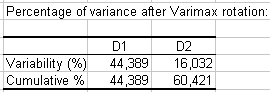The next results we want to look at, are the factor loadings after the varimax rotation. These results are used to interpret the meaning of the (rotated) factors.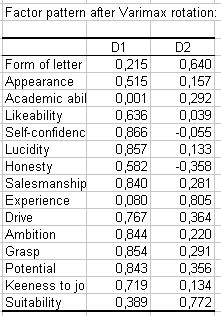From this table we can see that the first factor is highly positively related to Ambition, Self-confidence, Salesmanship and Lucidity. The second factor is loaded on Form of letter, Experience, and Suitability.

From these results, we can understand that the individuals that have high scores on the first factor are promising salesmen, while for other jobs such as management, individuals with high coordinates on the second and third factors might be more appropriate.

The following chart gives the position of the variables on axes F1 and F2. Other charts mixing other factors can be displayed.The following table gives the factor scores after the varimax rotation, which are the estimated coordinates of the observations on the factor axes.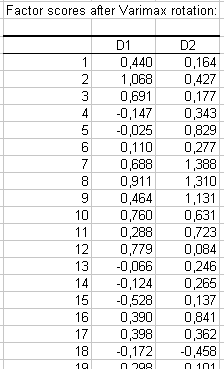XLSTAT displays the 2D maps on the selected factors. The chart below represents the map for F1 and F2.The following video addresses Factor Analysis with an illustration using XLSTAT.

### Contact Us

#### Contact our technical support team: support@xlstat.com

https://cdn.desk.com/
false
desk
Loading
seconds ago
a minute ago
minutes ago
an hour ago
hours ago
a day ago
days ago
about
false
Invalid characters found
/customer/portal/articles/autocomplete
9283